# Martin's Blog

## The Masser-Wüstholz isogeny theorem

Posted by Martin Orr on Wednesday, 25 April 2012 at 14:09

Let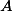and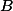be two isogenous abelian varieties over a number field. Can we be sure that there is an isogeny between them of small degree, where "small" is an explicit function ofand? In particular, our bound should not depend on; this means that the bound will imply Finiteness Theorem I, and hence the Shafarevich, Tate and Mordell conjectures.

The Masser-Wüstholz isogeny theorem answers this question, at least subject to a minor condition on polarisations (I think that this was removed in a later paper of Masser and Wüstholz but it is not too important anyway -- when deducing Finiteness Theorem I you can remove the polarisation issue with Zarhin's Trick).

Theorem. (Masser, Wüstholz 1993) Letandbe principally polarised abelian varieties over a number field. Suppose that there exists some isogeny. Then there is an isogenyof degree at most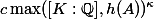whereandare constants depending only on the dimension of.

We will prove this using the Masser-Wüstholz period theorem which I discussed last time.

Read more...

## The Masser-Wüstholz period theorem

Posted by Martin Orr on Friday, 30 March 2012 at 12:20

I wanted to write a post about the Masser-Wüstholz isogeny theorem, which gives a quantitative version of Finiteness Theorem I. But it turned out to be too long so for today I will focus on the main ingredient in the proof of the isogeny theorem: the Masser-Wüstholz period theorem.

The period theorem gives a bound for the degree of the smallest abelian subvariety of a fixed abelian varietyhaving a given period ofin its tangent space. In this post I will explain the statement of the period theorem, in particular defining the degree of a (polarised) abelian variety, and give some properties of the degree which will be used in the proof of the isogeny theorem.

Period Theorem. (Masser, Wüstholz 1993) Letbe an abelian variety defined over a number fieldwith a principal polarisation. For any non-zero periodof, the smallest abelian subvarietyofwhose tangent space containssatisfieswhereandare constants depending only on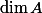.

Masser and Wüstholz gave a value forofwhere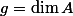. For myself, I am only interested in the existence of such a bound, but work has been done on improving it. If I correctly understand a recent preprint of Gaudron and Rémond, they show that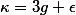suffices.

Read more...

## The Faltings height and normed modules

Posted by Martin Orr on Saturday, 31 December 2011 at 15:31

In this post I shall give the definition of the Faltings height of an abelian variety over any number field. Last time we did this over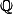only, and we used two properties of: the integers are a PID and there is only one archimedean place. To do things more generally, we will introduce the technology of normed modules and their degrees.

Read more...

## The Faltings height of an abelian variety over the rationals

Posted by Martin Orr on Thursday, 17 November 2011 at 15:58

The Faltings height is a real number attached to an abelian variety (defined over a number field), which is at the centre of Faltings' proof of Finiteness Theorem I. In this post all I will do is define the Faltings height of an abelian variety over, as already this requires a lot of preliminaries on cotangent and canonical sheaves of schemes. Further complications arise over other base fields, which I will discuss next time.

For an abelian varietyover, the Faltings height is the (logarithm of the) volume ofas a complex manifold with respect to a particular volume form, chosen using the-structure of. The preliminaries are needed in order to choose the volume form.

Faltings' proof of Finiteness I proceeds by showing that for any fixed number field, there are finitely many abelian varieties of bounded Faltings height. This is done by showing that the Faltings height is not far away from the classical height of a point representing the abelian variety in the moduli space. Then he shows that the Faltings height is bounded within an isogeny class. Both of these parts are difficult.

Read more...

## Finiteness theorems for abelian varieties

Posted by Martin Orr on Monday, 19 September 2011 at 16:34

Faltings famously proved the Mordell, Shafarevich and Tate conjectures in 1983. In this post I will discuss the relationships between the Tate and Shafarevich conjectures and some other finiteness theorems for abelian varieties.

Everything which I call a conjecture in this post is known to be true: they all follow from Finiteness Theorem I. Proving Finiteness Theorem I was the bulk of Faltings' work, but I am not going to talk about that today.

Finiteness Theorem I. Given a number fieldand an abelian varietydefined over, there are only finitely many isomorphism classes of abelian varieties defined overand isogenous to.

Read more...